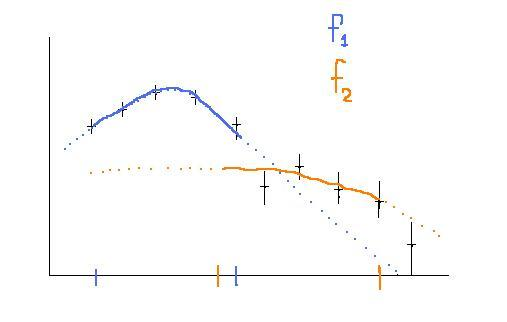# Trying several fits with only 2 functions?

• A
Gold Member
Well I was reading this paper http://inspirehep.net/record/1409825
and came across this comment:
The simulated top quark and diboson samples as well as the data-driven background estimate are statistically limited at large $m_T$. Therefore the expected number of events is extrapolated into the high $m_T$ region using fits. Several fits are carried out, exploring various fit ranges as well as the two fit functions $f(m_T) = e^{-a} m^b_T m_T^{c \log m_T}$ and $f(m_T) = \frac{a}{(m_T + b)^{c}}$. The fit with the best $\chi^2 /\text{d.o. f.}$ is used as the extrapolated background contribution, with an uncertainty evaluated using the RMS of all attempted fits

My question is basically a statistical one.... how can you make several fits using only 2 fitting functions?
Or do they mean something like fitting with func1 in some ranges [a,b] with a,b varying?
Also I do not understand well the uncertainty evaluation using the RMS of all attempted fits [how it works statistically].

Mentor
Or do they mean something like fitting with func1 in some ranges [a,b] with a,b varying?
That's how I would interpret the different fit ranges they tested.
Also I do not understand well the uncertainty evaluation using the RMS of all attempted fits [how it works statistically].
For a given m_T, you look at the average and RMS of all the values the different fits have at that point.

Gold Member
For a given m_T, you look at the average and RMS of all the values the different fits have at that point.

hmm... even if those fits were made over a partially common range? eg [200,300] and [250,400] ?

Mentor
The fits are correlated, sure, but you are not studying the sensitivity to the data fluctuations here, only the sensitivity to the fit method.

Gold Member
well my problem mainly comes from such a sketch I did on the scratch (so sorry for the wriggles and so on)...Obviously trying to determine an error based on what values the f1 and f2 have in some ranges (at eg the blue's peak) can be problematic...especially if your best fit happens to be that of f1.

Last edited:
Mentor
Well, if the fits look like that, you are doing something wrong.